Chapter 4 Part 1 - AD,AS and Related Concepts

Economics Class 12
Macroeconomics

Aggregate Supply = National Income = Consumption + Savings

AS = Y = C + S

Explanation

Aggregate Supply = National Income

Now this Income is either Spent or Consumed

Hence, we can say that

Aggregate Supply = National Income = Consumption + Savings

AS = Y = C + S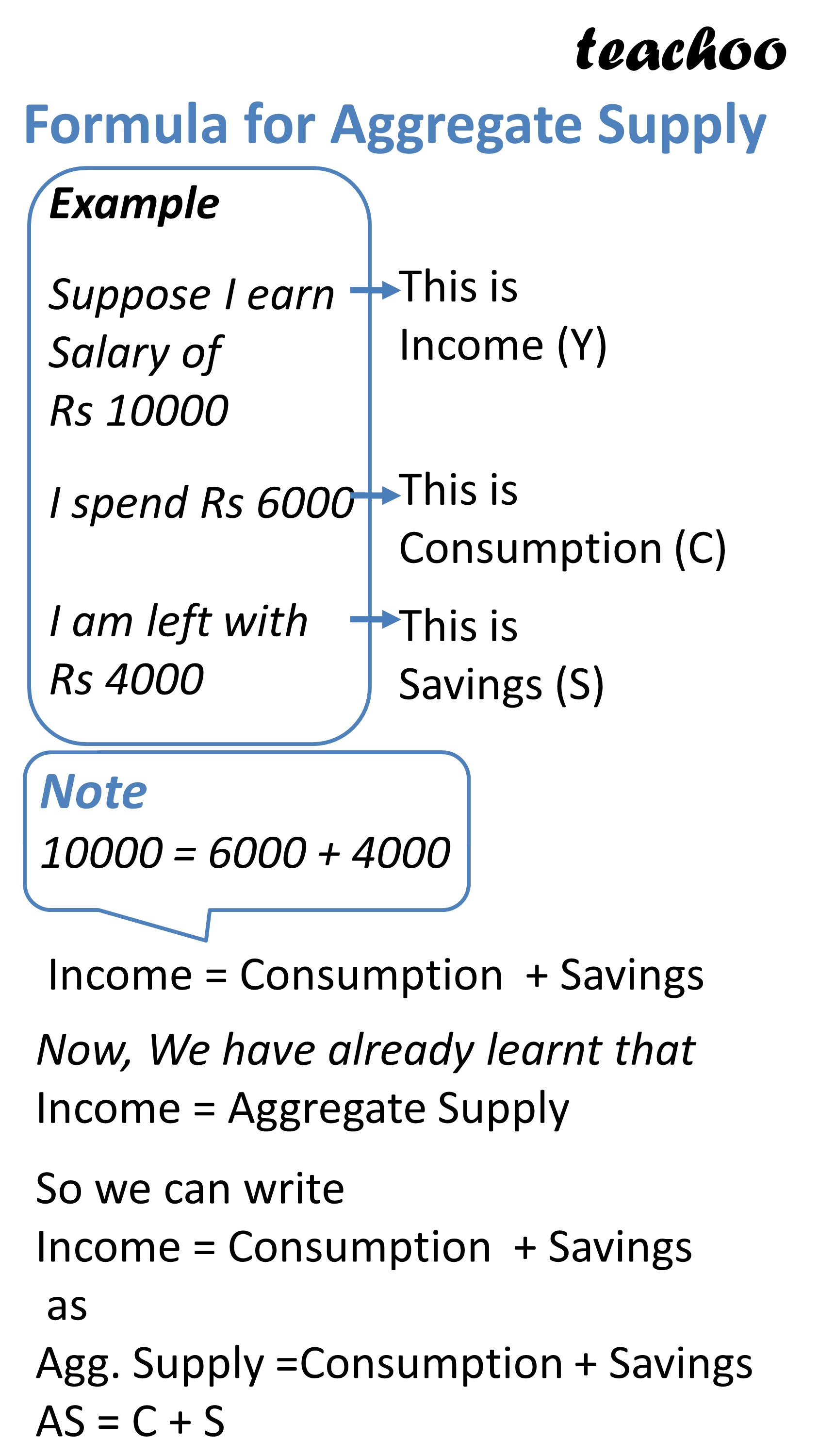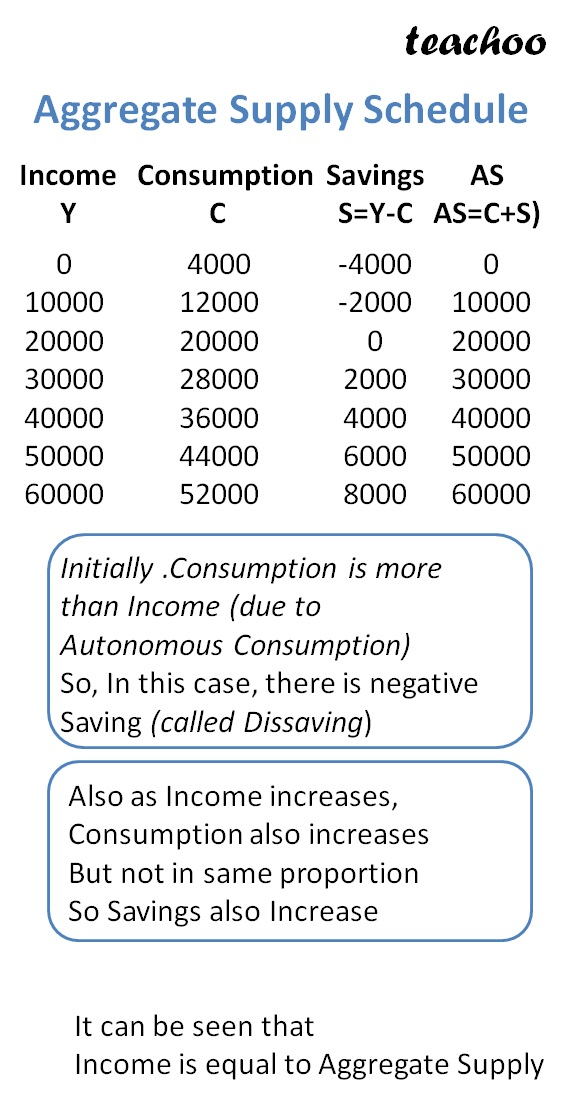Example

Lets suppose a person moves to a new city in search of job

In first month, he doesn’t find a job but has to pay 4000 on food

Hence, in first month

 Income Consumption Expenses Savings 0 4000 -4000

--> Person has to spend 4000 from his own pocket, so his savings are negative (Dissaving 4000)

Next month, person finds a job at a Salary of Rs 10000

He decides to spend 80% of his Income (Rs 4000 on food extra)

Hence, in second month

he spends 10000*80% + 4000 = 12000

 Income Consumption Expenses Savings 0 4000 -4000 10000 12000 -2000

--> Person has to spend 4000 from his own pocket, so his savings are negative (Dissaving 4000)

Next month, Person's income increases to 20000

He decides to spend 80% of his Income (Rs 4000 on food extra)

Hence, in this month

He spends 20000*80% + 4000 = 20000

 Income Consumption Expenses Savings 0 4000 -4000 10000 12000 -2000 20000 20000 0

Next to next month, Person's income increases to 30000

He decides to spend 80% of his Income (Rs 4000 on food extra)

Hence, in third month

Income = 30000*80% + 4000 = 24000 + 4000 = 28000

Savings = 30000 - 28000 = 2000

 Income Consumption Expenses Savings 0 4000 -4000 10000 12000 -2000 20000 20000 0 30000 28000 2000

Similarly his income exceed each year by 10000 and he continues to spend 80% of it and 4000 extra in following manner: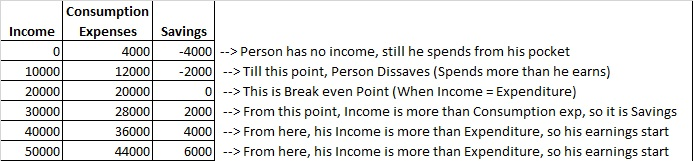## How to Make Graph for Aggregate Supply?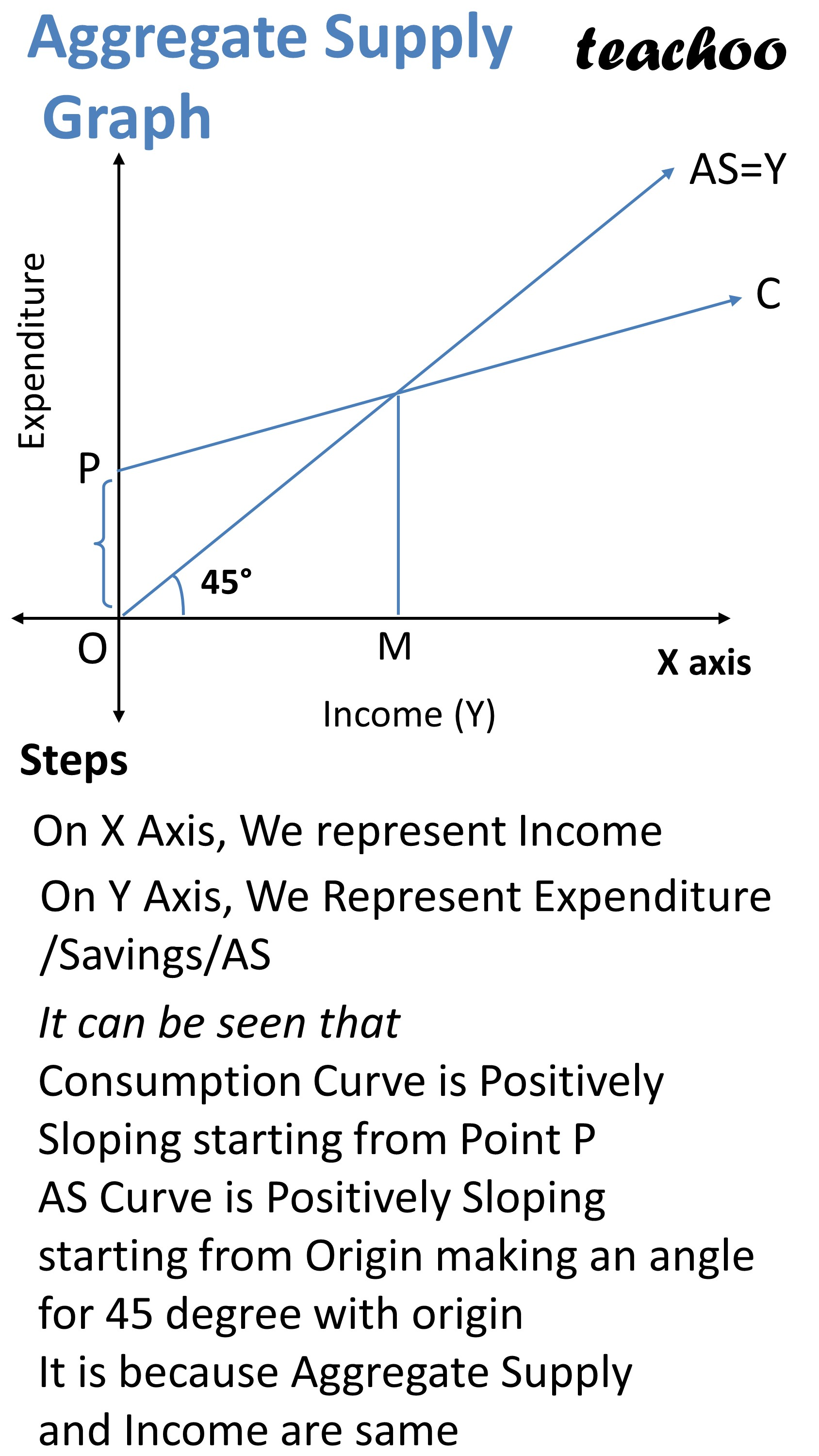Step 1 - We draw Consumption Curve

On X Axis, We represent Income

On Y Axis, We Represent Expenditure

It can be seen that

Consumption Curve C is Positively Sloping

(Increase with Increase in Income)

Consumption Curve starts from Point S (OS Representing Autonomous Consumption Expenditure)

Step 2 - Draw Aggregate Supply Curve

AS Curve is also positively Sloping

It Starts from Point 0

It forms a 45 degree line with Y axis

It is because Aggregate Supply and Income are same

Step 3 - Showing Savings and Dissavings

What are Savings?

Income = Consumption + Savings

Since, Income = Aggregate Supply, we can also say that

Aggregate Supply = Consumption + Savings

AS = C + S

S = AS - C

Hence, Savings are Difference between Aggregate Suppy and Consumption expenditure

## What are Dissavings?

Negative Savings are called dissavings

It happens when Aggregate Supply is less than Consumption expenditure

Disaavings = C - AS

## How are Savings and Dissavings shown on Graph?

It is shown as difference between AS Curve and Consumption Curve

Point where AS Curve and Consumption curve intersect is called Break even Point (Zero Savings)

Before Break even point, difference between 2 curves is Dissavings

After break even point, difference between 2 curves is Savings

Hence, Savings is difference of Aggregate Supply Curve and Consumption Curve

In the initial stage,

AS is more than C

Hence, it is Dissaving's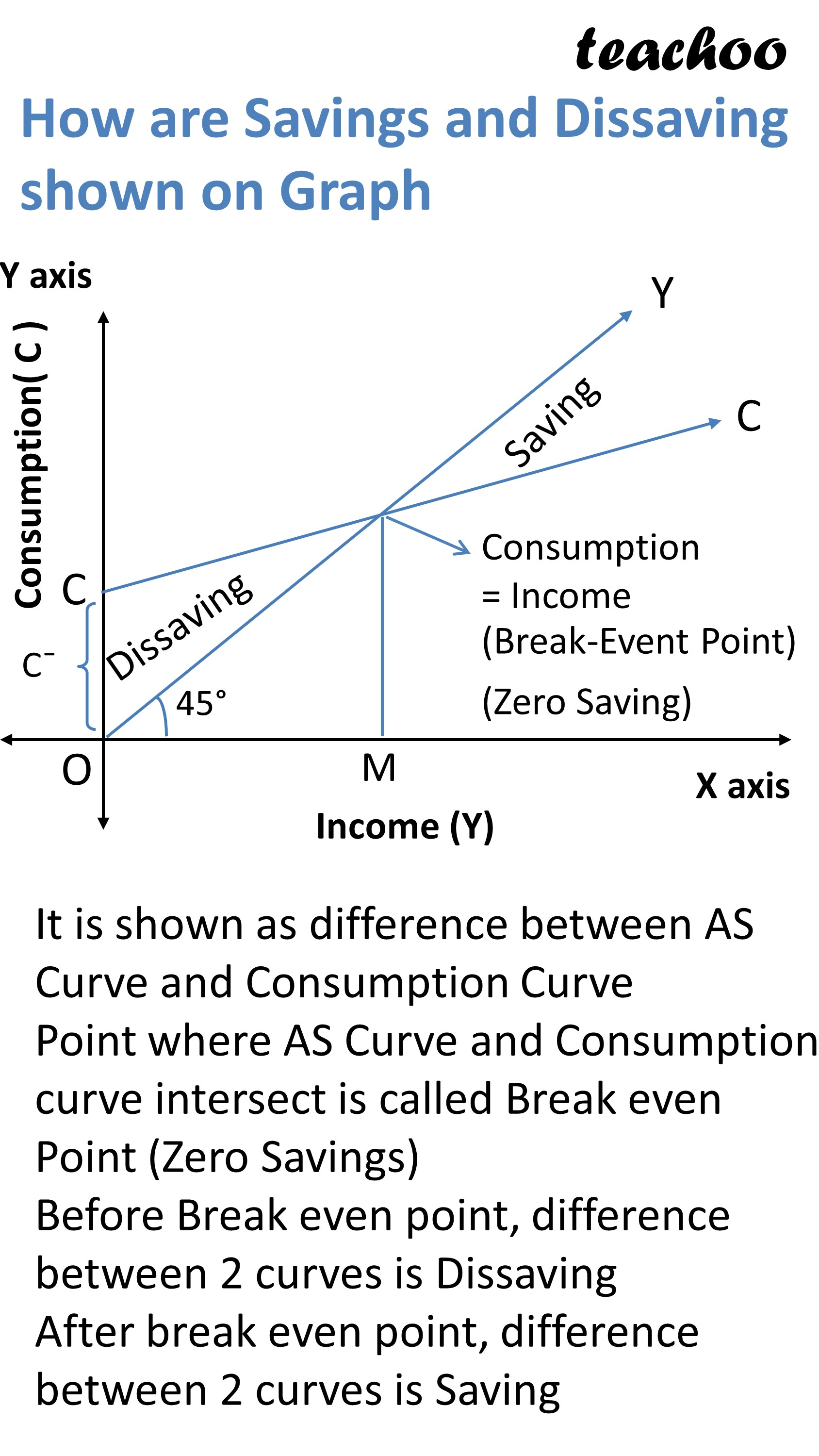## How to Get Savings Curve from Consumption Curve?

We know that at 0 level of income, still there is some consumption

As consumption curve starts at Point 0 (OC is amt of autonomous consumption)

At same level of income, Savings will be Negative

Suppose my Income is 0, Consumption is 3000

Hence there will be Savings of -3000

Hence Savings curve start from point S such that

OD = OS

Now we know that at break even point E

Consumption = Income

At this point Consumption and Saving curve intersect

Now at this point, Savings will be 0

Hence Savings curve will lie at x axis

We name this point R

Hence if we connect S and R, we get Savings Curve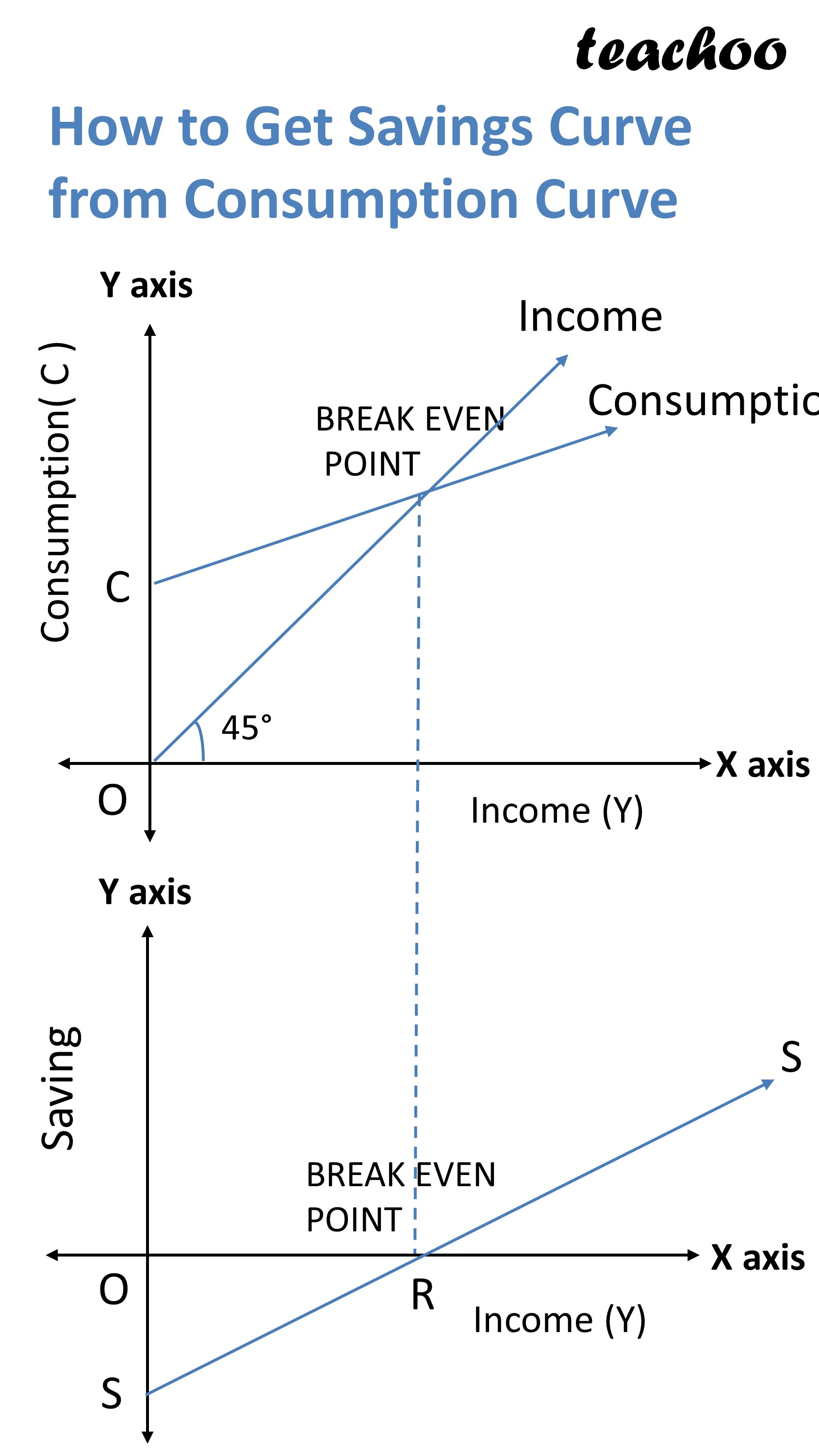### NCERT Questions

No questions in this part

### Other Books

#### Question 1

How to Get Savings Curve from Consumption Curve?

Get live Maths 1-on-1 Classs - Class 6 to 12

### Transcript

Formula for Aggregate Supply Example Suppose I earn Salary of Rs 10000 I spend Rs 6000 I am left with Rs 4000 This is Income (Y) This is Consumption (C) This is Savings (S) Note 10000 = 6000 + 4000 Income = Consumption + Savings Now, We have already learnt that Income = Aggregate Supply So we can write Income = Consumption + Savings as Agg. Supply =Consumption + Savings AS = C + S Aggregate Supply Schedule Income Y 0 10000 20000 30000 40000 50000 60000 Consumption C 4000 12000 20000 28000 36000 44000 52000 Savings S=Y-C -4000 -2000 0 2000 4000 6000 8000 AS AS=C+S) 0 10000 20000 30000 40000 50000 60000 Initially .Consumption is more than Income (due to Autonomous Consumption) So, In this case, there is negative Saving (called Dissaving) Also as Income increases, Consumption also increases But not in same proportion So Savings also Increase It can be seen that Income is equal to Aggregate Supply Aggregate Supply Graph Steps On X Axis, We represent Income On Y Axis, We Represent Expenditure /Savings/AS It can be seen that Consumption Curve is Positively Sloping starting from Point P AS Curve is Positively Sloping starting from Origin making an angle for 45 degree with origin It is because Aggregate Supply and Income are same How are Savings and Dissaving shown on Graph It is shown as difference between AS Curve and Consumption Curve Point where AS Curve and Consumption curve intersect is called Break even Point (Zero Savings) Before Break even point, difference between 2 curves is Dissaving After break even point, difference between 2 curves is Saving How to Get Savings Curve from Consumption Curve